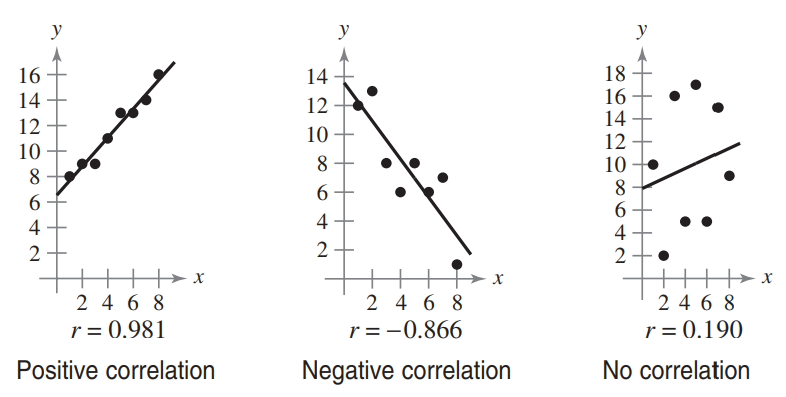Chapter 7.7, Problem 20E### Calculus: An Applied Approach (Min...

10th Edition
Ron Larson
ISBN: 9781305860919

#### Solutions

Chapter
Section### Calculus: An Applied Approach (Min...

10th Edition
Ron Larson
ISBN: 9781305860919
Textbook Problem
1 views

# Determining Correlation In Exercises 19-24, plot the points and determine whether the data have positive, negative, or no linear correlation (see figures below). Then use a graphing utility to find the value of r and confirm your result. The number r is called the correlation coefficient. It is a measure of how well the model fits the data. Correlation coefficients vary between -1 and 1, and the closer |r| is to 1, the better the model.( 1 , 7.5 ) , ( 2 , 7 ) , ( 3 , 7 ) , ( 4 , 6 ) , ( 5 , 5 ) , ( 6 , 4.9 )

To determine

To graph: The points (1,7.5),(2,7),(3,7),(4,6),(5,5),(6,4.9) and determine whether these points have positive, negative or no linear correlation. Also determine the correlation coefficient using graphing utility.

Explanation

Given Information:

The provided points are (1,7.5),(2,7),(3,7),(4,6),(5,5),(6,4.9).

Graph:

Consider the provided points,

(1,7.5),(2,7),(3,7),(4,6),(5,5),(6,4.9)

Label these points on coordinate axis and connect them with a straight line as shown below,

It can be observed from the graph that the graph has negative correlation

### Still sussing out bartleby?

Check out a sample textbook solution.

See a sample solution

#### The Solution to Your Study Problems

Bartleby provides explanations to thousands of textbook problems written by our experts, many with advanced degrees!

Get Started

#### Convert the expressions in Exercises 6584 to power form. x2y

Finite Mathematics and Applied Calculus (MindTap Course List)

#### (rn)4r52n

Applied Calculus for the Managerial, Life, and Social Sciences: A Brief Approach

#### In problems 23-58, perform the indicated operations and simplify. 30.

Mathematical Applications for the Management, Life, and Social Sciences

#### True or False: n=1(1)nnn+3 converges.

Study Guide for Stewart's Single Variable Calculus: Early Transcendentals, 8th